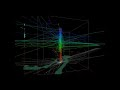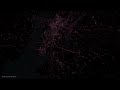Page 1 of 1 (6 posts)

• pyprocessing

#### Tags

Last update:
Wed Nov 13 17:10:04 2019

# City flows unfolding with the other Processing

A previous version of this post has been published in German on Die bemerkenswerte Karte.

Visualizations of mobility data such as taxi or bike sharing trips have become very popular. One of the best most recent examples is cf. city flows developed by Till Nagel and Christopher Pietsch at the FH Potsdam. cf. city flows visualizes the rides in bike sharing systems in New York, Berlin and London at different levels of detail, from overviews of the whole city to detailed comparisons of individual stations:

The visualizations were developed using Unfolding, a library to create interactive maps and geovisualizations in Processing (the other Processing … not the QGIS Processing toolbox) and Java. (I tinkered with the Python port of Processing in 2012, but this is certainly on a completely different level.)

The insights into the design process, which are granted in the methodology section section of the project website are particularly interesting. Various approaches for presenting traffic flows between the stations were tested. Building on initial simple maps, where stations were connected by straight lines, consecutive design decisions are described in detail:

The results are impressive. Particularly the animated trips convey the dynamics of urban mobility very well:

However, a weak point of this (and many similar projects) is the underlying data. This is also addressed directly by the project website:

Lacking actual GPS tracks, the trip trajectories are rendered as smooth paths of the calculated optimal bike routes

This means that the actual route between start and drop off location is not known. The authors therefore estimated the routes using HERE’s routing service. The visualization therefore only shows one of many possible routes. However, cyclists don’t necessarily choose the “best” route as determined by an algorithm – be it the most direct or otherwise preferred. The visualization does not account for this uncertainty in the route selection. Rather, it gives the impression that the cyclist actually traveled on a certain route. It would therefore be undue to use this visualization to derive information about the popularity of certain routes (for example, for urban planning). Moreover, the data only contains information about the fulfilled demand, since only trips that were really performed are recorded. Demand for trips which could not take place due to lack of bicycles or stations, is therefore missing.

As always: exercise some caution when interpreting statistics or visualizations and then sit back and enjoy the animations.

If you want to read more about GIS and transportation modelling, check out
Loidl, M.; Wallentin, G.; Cyganski, R.; Graser, A.; Scholz, J.; Haslauer, E. GIS and Transport Modeling—Strengthening the Spatial Perspective. ISPRS Int. J. Geo-Inf. 2016, 5, 84. (It’s open access.)# Space-Time Cubes – Exploring Twitter Streams III

This post continues my quest of exploring the spatial dimension of Twitter streams. I wanted to try one of the classic spatio-temporal visualization methods: Space-time cubes where the vertical axis represents time while the other two map space. Like the two previous examples, this visualization is written in pyprocessing, a Python port of the popular processing environment.This space-time cube shows twitter trajectories that contain at least one tweet in New York Times Square. The 24-hour day starts at the bottom of the cube and continues to the top. Trajectories are colored based on the time stamp of their start tweet.

Additionally, all trajectories are also drawn in context of the coastline (data: OpenStreetMap) on the bottom of the cube.

While there doesn’t seem to be much going on in the early morning hours, we can see quite a busy coming and going during the afternoon and evening. From the bunch of vertical lines over Times Square, we can also assume that some of our tweet authors spent a considerable time at and near Times Square.

I’ve also created an animated version. Again, I recommend to watch it in HD.# Pyprocessing for 3D Animations

I’ve been looking into the 3D capabilities of Pyprocessing for the creation of animated space-time cubes.

There are subtle differences between Processing and Pyprocessing. Processing is documented pretty well but I prefer Python over Java any time. So here is my port of the Processing “Cubes within Cube” example as a reference for how 3D animations are done in Pyprocessing.(You can watch the animation live on the Processing site.)

```from pyprocessing import *
from random import random

cubies = 20
c = *cubies

# Controls cubie's movement
x = [0.0]*cubies
y = [0.0]*cubies
z = [0.0]*cubies
xSpeed = [0.0]*cubies
ySpeed = [0.0]*cubies
zSpeed = [0.0]*cubies

# Controls cubie's rotation
xRot = [0.0]*cubies
yRot = [0.0]*cubies
zRot = [0.0]*cubies

stage = None

# Size of external cube
bounds = 300.0

def setup():
size(640, 360);

for i in range(0,cubies):
# Each cube face has a random color component

# Cubies are randomly sized
cubieSize = random()*10+5
c[i] =  Cube(cubieSize, cubieSize, cubieSize)

# Initialize cubie's position, speed and rotation
x[i] = 0.0
y[i] = 0.0
z[i] = 0.0

xSpeed[i] = random()*4-2
ySpeed[i] = random()*4-2
zSpeed[i] = random()*4-2

xRot[i] = random()*60+40
yRot[i] = random()*60+40
zRot[i] = random()*60+40

def draw():
background(50)
lights()

# Center in display window
translate(width/2, height/2, -130)

# Outer transparent cube
noFill()

# Rotate everything, including external large cube
rotateX(frame.count * 0.001)
rotateY(frame.count * 0.002)
rotateZ(frame.count * 0.001)
stroke(255)

# Draw external large cube
stage =  Cube(bounds, bounds, bounds);
stage.create()

# Move and rotate cubies
for i in range(0,cubies):
pushMatrix()
translate(x[i], y[i], z[i])
rotateX(frame.count*PI/xRot[i])
rotateY(frame.count*PI/yRot[i])
rotateX(frame.count*PI/zRot[i])
noStroke()
x[i] += xSpeed[i]
y[i] += ySpeed[i]
z[i] += zSpeed[i]
popMatrix()

# Draw lines connecting cubbies
stroke(0)
if i < cubies-1:
line(x[i], y[i], z[i], x[i+1], y[i+1], z[i+1])

# Check wall collisions
if x[i] > bounds/2 or x[i] < -bounds/2:
xSpeed[i]*=-1

if y[i] > bounds/2 or y[i] < -bounds/2:
ySpeed[i]*=-1

if z[i] > bounds/2 or z[i] < -bounds/2:
zSpeed[i]*=-1

# Custom Cube Class

class Cube():
def __init__(self,w,h,d):
self.vertices = *24
self.w = w;
self.h = h;
self.d = d;

# cube composed of 6 quads
#front
self.vertices =  PVector(-w/2,-h/2,d/2)
self.vertices =  PVector(w/2,-h/2,d/2)
self.vertices =  PVector(w/2,h/2,d/2)
self.vertices =  PVector(-w/2,h/2,d/2)
#left
self.vertices =  PVector(-w/2,-h/2,d/2)
self.vertices =  PVector(-w/2,-h/2,-d/2)
self.vertices =  PVector(-w/2,h/2,-d/2)
self.vertices =  PVector(-w/2,h/2,d/2)
#right
self.vertices =  PVector(w/2,-h/2,d/2)
self.vertices =  PVector(w/2,-h/2,-d/2)
self.vertices =  PVector(w/2,h/2,-d/2)
self.vertices =  PVector(w/2,h/2,d/2)
#back
self.vertices =  PVector(-w/2,-h/2,-d/2)
self.vertices =  PVector(w/2,-h/2,-d/2)
self.vertices =  PVector(w/2,h/2,-d/2)
self.vertices =  PVector(-w/2,h/2,-d/2)
#top
self.vertices =  PVector(-w/2,-h/2,d/2)
self.vertices =  PVector(-w/2,-h/2,-d/2)
self.vertices =  PVector(w/2,-h/2,-d/2)
self.vertices =  PVector(w/2,-h/2,d/2)
#bottom
self.vertices =  PVector(-w/2,h/2,d/2)
self.vertices =  PVector(-w/2,h/2,-d/2)
self.vertices =  PVector(w/2,h/2,-d/2)
self.vertices =  PVector(w/2,h/2,d/2)

# Draw cube
for i in range(0,6):
for j in range(0,4):
vertex(self.vertices[j+4*i].x, self.vertices[j+4*i].y, self.vertices[j+4*i].z)
endShape()

run()
```# A Visual Exploration of Twitter Streams II

After my first shot at analyzing Twitter data visually I received a lot of great feedback. Thank you!

For my new attempt, I worked on incorporating your feedback such as: filter unrealistic location changes, show connections “grow” instead of just popping up and zoom to an interesting location. The new animation therefore focuses on Manhattan – one of the places with reasonably high geotweet coverage.

The background is based on OpenStreetMap coastline data which I downloaded using QGIS OSM plugin and rendered in pyprocessing together with the geotweets. To really see what’s going on, switch to HD resolution and full screen:It’s pretty much work-in-progress. The animation shows similar chaotic patterns seen in other’s attempts at animating tweets. To me, the distribution of tweets looks reasonable and many of the connection lines seem to actually coincide with the bridges spanning to and from Manhattan.

This work is an attempt at discovering the potential of Twitter data and at the same time learning some pyprocessing which will certainly be useful for many future tasks. The next logical step seems to be to add information about interactions between users and/or to look at the message content. Another interesting task would be to add interactivity to the visualization.# A Visual Exploration of Twitter Streams

Twitter streams are curious things, especially the spatial data part. I’ve been using Tweepy to collect tweets from the public timeline and what did I discover? Tweets can have up to three different spatial references: “coordinates”, “geo” and “place”. I’ll still have to do some more reading on how to interpret these different attributes.

For now, I have been using “coordinates” to explore the contents of a stream which was collected over a period of five hours using

`stream.filter(follow=None,locations=(-180,-90,180,90))`

for global coverage. In the video, each georeferenced tweet produces a new dot on the map and if the user’s coordinates change, a blue arrow is drawn:While pretty, these long blue arrows seem rather suspicious. I’ve only been monitoring the stream for around five hours. Any cross-Atlantic would take longer than that. I’m either misinterpreting the tweets or these coordinates are fake. Seems like it is time to dive deeper into the data.# Listing Available Fonts For Pyprocessing

Today’s post is a short note-to-self.

This script lists available fonts and renders a small preview using Tkinter and pyprocessing.

```from pyprocessing import *
import Tkinter
import tkFont

t = Tkinter.Toplevel() # without root window the following line fails
fonts = tkFont.families()
t.destroy()

size(1500,900)
fill(0)
rect(0,0,1500,900)
fill(255)

fontsize=14
lineheight=fontsize*1.2
y=lineheight
x=0

for font_name in fonts:
print font_name
font = createFont(font_name, fontsize)
textFont(font)
text("Hello world!   ("+font_name+")", x,y,1000,66)
y+=lineheight
if y >= 900:
x+=500
y=lineheight

run()
```On the TODO list:

• Find out how to turn these fonts bold or italics.• Page 1 of 1 ( 6 posts )
• pyprocessing